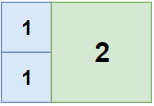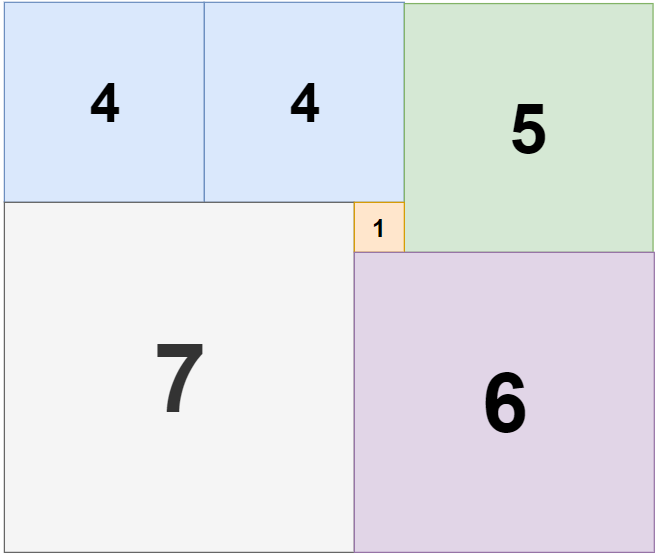# 铺瓷砖

## 1240. 铺瓷砖 (Hard)```输入：n = 2, m = 3

`解释：3` 块地砖就可以铺满卧室。
`     2` 块 `1x1 地砖`
`     1` 块 `2x2 地砖```````输入：n = 5, m = 8

``````输入：n = 11, m = 13

```

• `1 <= n <= 13`
• `1 <= m <= 13`

[动态规划] [回溯算法]